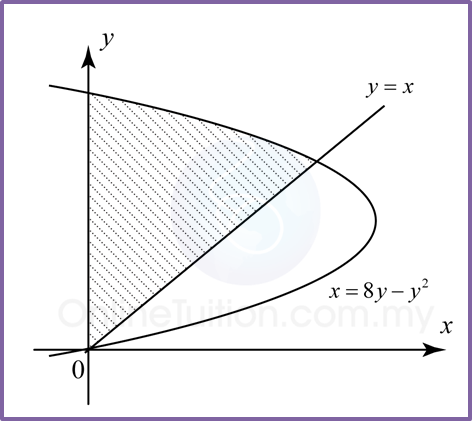# 3.5 Integration as the Summation of Areas

3.5 Integration as the Summation of Areas

(A) Area of the region between a Curve and the x-axis.Area of the shaded region;  $A={\int }_{a}^{b}y\text{}dx$

(B) Area of the region between a curve and the y-axis.Area of the shaded region;  $A={\int }_{a}^{b}x\text{}dy$

(C) Area of the region between a curve and a straight line.Area of the shaded region;  $A={\int }_{a}^{b}f\left(x\right)\text{}dx-{\int }_{a}^{b}g\left(x\right)\text{}dx$

Example 1
Find the area of the shaded region.Solution:
$\begin{array}{l}\text{Area of the shaded region}\\ ={\int }_{a}^{b}y\text{}dx\\ ={\int }_{0}^{4}\left(6x-{x}^{2}\right)dx\\ ={\left[\frac{6{x}^{2}}{2}-\frac{{x}^{3}}{3}\right]}_{0}^{4}\\ =\left[3{\left(4\right)}^{2}-\frac{{\left(4\right)}^{3}}{3}\right]-0\\ =26\frac{2}{3}{\text{unit}}^{2}\end{array}$

Example 2
Find the area of the shaded region.Solution:
y = x —–(1)
x = 8yy2—–(2)
Substitute (1) into (2),
y = 8yy2
y2 – 7y = 0
y (y – 7) = 0
y = 0 or 7
From (1), x = 0 or 7
Therefore the intersection points of the curve and the straight line is (0, 0) and (7, 7).

Intersection point of the curve and y-axis is,
x = 8yy2
At y-axis, x = 0
0 = 8yy2
y (y – 8) = 0
y = 0, 8

Area of shaded region = (A1) Area of triangle + (A2) Area under the curve from y = 7 to y = 8.
$\begin{array}{l}=\frac{1}{2}×\text{base}×\text{height +}{\int }_{7}^{8}x\text{}dy\\ =\frac{1}{2}×\left(7\right)\left(7\right)+{\int }_{7}^{8}\left(8y-{y}^{2}\right)\text{}dy\\ =\frac{49}{2}+{\left[\frac{8{y}^{2}}{2}-\frac{{y}^{3}}{3}\right]}_{7}^{8}\\ =24\frac{1}{2}+\left[4{\left(8\right)}^{2}-\frac{{\left(8\right)}^{3}}{3}\right]-\left[4{\left(7\right)}^{2}-\frac{{\left(7\right)}^{3}}{3}\right]\\ =24\frac{1}{2}+85\frac{1}{3}-81\frac{2}{3}\\ =28\frac{1}{6}{\text{unit}}^{2}\end{array}$